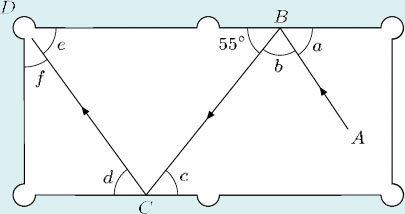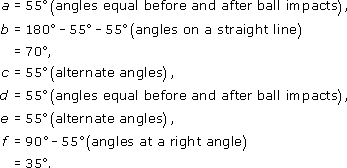Science, Maths & Technology

### Become an OU studentGeometry

Start this free course now. Just create an account and sign in. Enrol and complete the course for a free statement of participation or digital badge if available.

# Try some yourself

## Question 1

Find α and β in each of the diagrams below.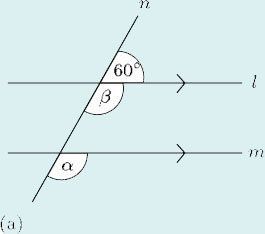(a) Now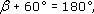so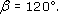But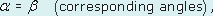so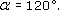## Question 2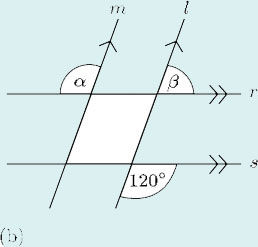(b)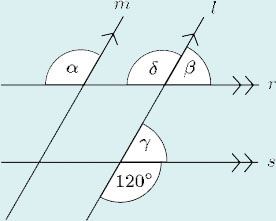There are many ways of finding the sizes of these angles. This is only one of them:

As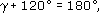it follows that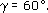But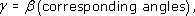so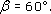Similarly,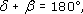so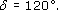But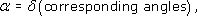so## Question 3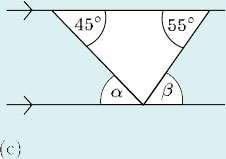(c) α = 45° (alternate angles).

β = 55° (alternate angles).

## Question 4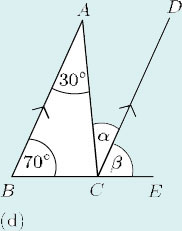(d) α =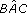= 30° (alternate angles).

β =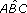= 70° (corresponding angles).

## Question 5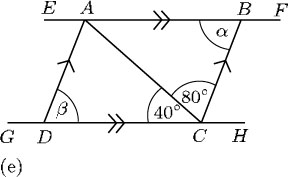(e) As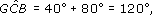it follows that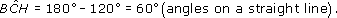But β and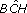are corresponding angles, soWhereas α andare alternate angles, so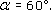## Question 6

2 This diagram shows part of some bannister rails. The handrail makes an angle of 40° with the horizontal. Calculate angles α, β, γ and δ.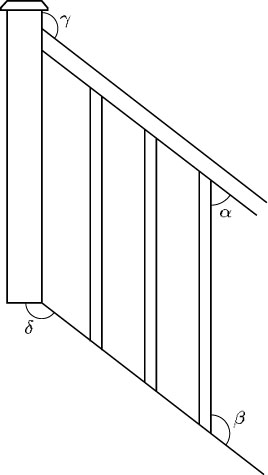It is a good idea to sketch a diagram, adding some horizontal lines where necessary.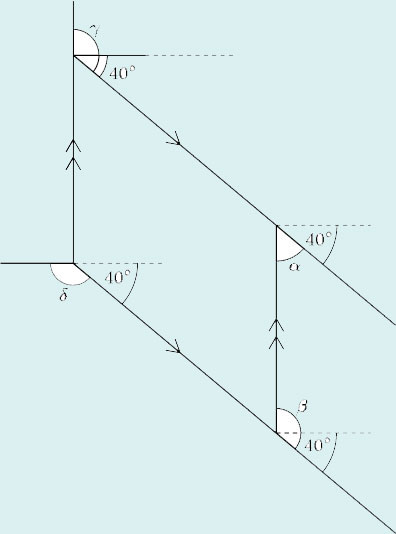Assume the lines marked are pairs of parallel lines. Then, since the handrail makes an angle of 40° with the horizontal,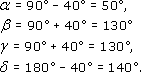## Question 7

3 The arrows on the diagram below indicate the idealised path (ABCD) of a snooker ball on a snooker table. Assume that the angles between the cushion (the edge of the snooker table) and the path of the ball before and after it impacts with the cushion are equal. Calculate the sizes of the angles marked a, b, c, d, e and f.